This Mathcad file demonstrates how to calculate the discrete fourier transform (DFT) by formula.
Step 1: Define sampling frequency (multiple of 2) and build a waveform with several component frequencies.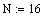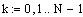N samples per cycle
Amplitudes, phase angles, and harmonic numbers of component frequencies.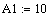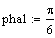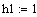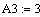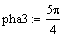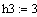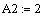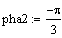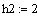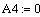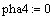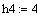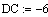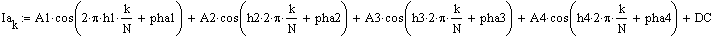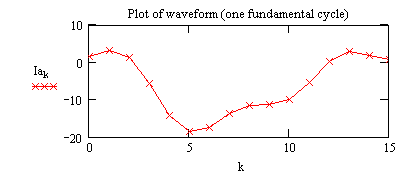Step 2: Perform the DFT calculation, resulting in a real and imaginary vector.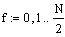Compute the real and imaginary DFT vectors.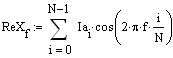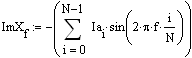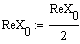Scale the magnitude of the end frequency bins of the real DFT vector.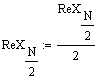Calculate magnitude of component frequencies in the DFT and scale properly.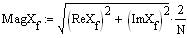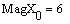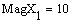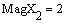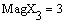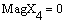Display magnitudes, should equal what was input
in the creation of the original waveform.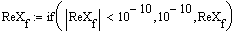Get rid of zeros if present to allow arctan calculation (avoid zero in denominator).
Compute phase angles and zero those that
are very near zero for clean display.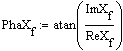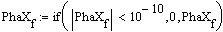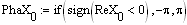Correct phase angle of zero frequency component (DC).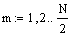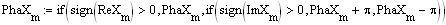Correct results of arctan computation
of frequencies above zero.
Step 4: Rebuild the waveform by using the results of the DFT (magnitude and phase angle of the component frequencies).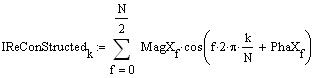Rebuild waveform from results of DFT.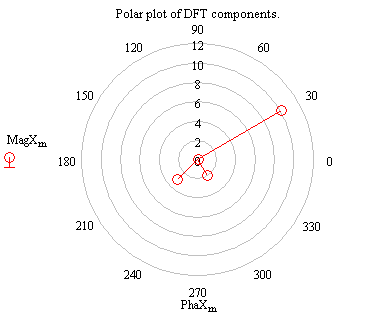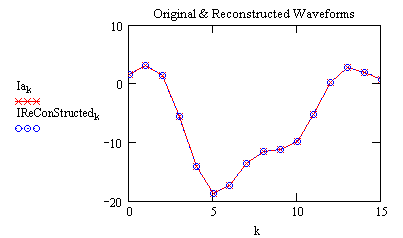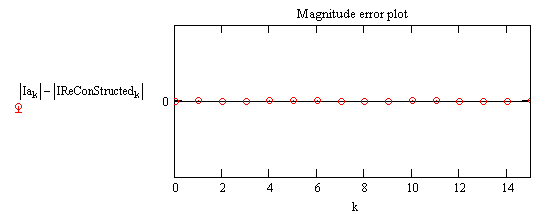Russell W. Patterson, P.E.
Manager, System Protection & Analysis
Tennessee Valley Authority
rwpatterson@tva.gov

Reference: "The Scientist and Engineer's Guide to Digital Signal Processing" by Steven W. Smith
ISBN: 0-9660176-3-3, Website: www.DSPguide.com